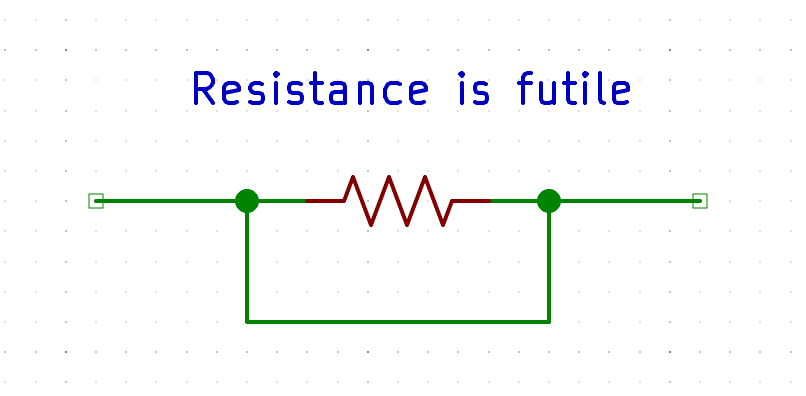# Layout view of resistors

edited March 2021 in Layout

Hello, I am trying to learn the concepts in LVS and device extraction. I am using an LVS script to automatically generate netlists.
I have a basic question. I don't understand why the resistance reduces to half even though I connect the resistors in series (or at least I think I connect them in series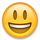. But it looks like they are connected parallel)

The following layouts consist of two resistors. One of them has separate and the other has connected resistors. When I produce the netlist you see the resistance values as 10.32 and 5.16. I don't understand why resistance reduces to half when I connect these two resistors. Shouldn't they become a single resistor with resistance doubled (since they are connected in series. -or am I wrong? Are they actually parallel?)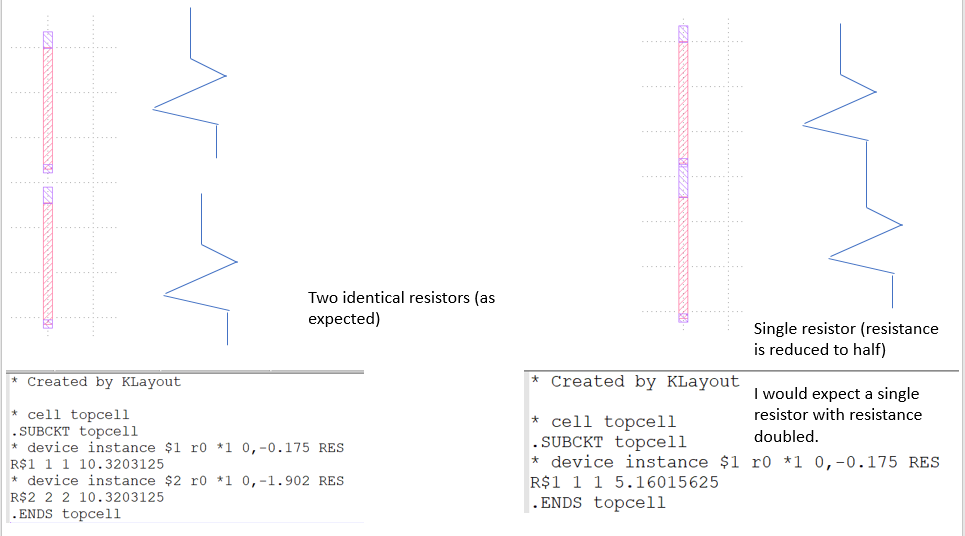If you can make an explanation it would be really helpful! (By the way is there a reference (book etc. ) where I can check how basic electronic components in layout view look like? (Instead of the logical view)

Thanks!

PS: This is the LVS code I use to automatically generate netlists

``````target_netlist("resistor2.cir", write_spice, "Created by KLayout")

deep

# Produce LVS report
report_lvs

res_layer = input(1,0)
contact_layer = input(2,0)

bulk = polygon_layer

sheet_rho = 1
model_name = "RES"
extract_devices(resistor(model_name, sheet_rho), { "R" => res_layer, "C" => contact_layer })

connect(res_layer,contact_layer)
#connect_global(bulk, "SUBSTRATE")
report_netlist("resistor.l2n")
# Netlist normalization
netlist.simplify
``````

• Whether they are series or parallel depends on
whether the endcaps are the same net or different.
The netlisting appears to think they are the same
net. But "why?" is probably the question.

Now there's something funny about the two
endcaps of both resistor segments, as if perhaps
the "longer" one is also contacting "something"
(NWell? Substrate? Or just a non-identical detail
layout?) as is the norm on implanted resistors
of single stripe - one contact also grabs the
isolation region. Then if the isolation is treated
as a "local global net" (i.e. extraction logic calls
it an ohmic short) than permute-parallel rules
(forget what klayout calls this) might give you
the outcome you're seeing.

• edited March 2021

Following and testing results are here code follow's
The test gds can be found here
https://github.com/hgroller/test_res

``````# Drawing layers
deep

nwell       = input(1, 0)
diff        = input(2, 0)
pplus       = input(3, 0)
nplus       = input(4, 0)
poly        = input(5, 0)
thickox     = input(6, 0)
polyres     = input(7, 0)
contact     = input(8, 0)
metal1      = input(9, 0)
via         = input(10, 0)
metal2      = input(11, 0)

# Special layer for bulk terminals

bulk        = make_layer

# Computed layers

poly_not_res  = poly - polyres
poly_in_res   = poly & polyres

diff_in_nwell = diff & nwell
pdiff       = diff_in_nwell - nplus
ntie        = diff_in_nwell & nplus
pgate       = pdiff & poly_not_res
psd         = pdiff - pgate
hv_pgate    = pgate & thickox
lv_pgate    = pgate - hv_pgate
hv_psd      = psd & thickox
lv_psd      = psd - thickox

diff_outside_nwell = diff - nwell
ndiff      = diff_outside_nwell - pplus
ptie       = diff_outside_nwell & pplus
ngate      = ndiff & poly_not_res
nsd        = ndiff - ngate
hv_ngate   = ngate & thickox
lv_ngate   = ngate - hv_ngate
hv_nsd     = nsd & thickox
lv_nsd     = nsd - thickox

# Resistor device extraction

class CustomResistorExtraction < RBA::GenericDeviceExtractor

def initialize(name, sheet_rho)
self.name = name
@sheet_rho = sheet_rho
end

def setup

define_layer("C", "Conductor")
define_layer("R", "Resistor")

register_device_class(RBA::DeviceClassResistor::new)

end

def extract_devices(layer_geometry)

# layer_geometry provides the input layers in the order they are
# defined with "define_layer"
# conductor is supposed to be "conductor outside marker"
# resistor is supposed to be "conductor inside marker"
conductor = layer_geometry
resistor  = layer_geometry

# the index of the conductor layer (needed to make the terminals)
conductor_geometry_index = 0

resistor_merged = resistor.merged

# this will be the edge where the resistor turns into conductor
marker_edges = conductor.merged.edges & resistor_merged.edges

resistor_merged.each do |r|

# identify the edges where this resistor shape ends
interface_edges = marker_edges.interacting(RBA::Region::new(r))

# form terminal shapes from these edges
terminals = interface_edges.extended_out(1)

if terminals.size != 2
error("Resistor shape does not touch marker border in exactly two places", r)
else

# A = L*W
# P = 2*(L+W)
# -> L = p+sqrt(p*p-A)
# -> W = p-sqrt(p*p-A)
# (p=P/4)

p = 0.25 * r.perimeter
a = r.area

d = Math.sqrt(p * p - a)
l = p + d
w = p - d

if w > 1e-3

device = create_device
device.set_parameter(RBA::DeviceClassResistor::PARAM_R, @sheet_rho * l / w);
define_terminal(device, RBA::DeviceClassResistor::TERMINAL_A, conductor_geometry_index, terminals);
define_terminal(device, RBA::DeviceClassResistor::TERMINAL_B, conductor_geometry_index, terminals);

end

end

end

end

def get_connectivity(layout, layers)

# the layer definition is marker, conductor, resistor
# * resistor is used for extraction
# * conductor is used for producing the terminals
conductor = layers
resistor  = layers
conn = RBA::Connectivity::new
conn.connect(resistor, resistor)
conn.connect(conductor, resistor)

return conn

end

end

# Resistor Definition
# Assumes a sheet rho of 150 Ohm/Square
#Define this as you like
res_ex = CustomResistorExtraction::new("RES", 1.0)
extract_devices(res_ex, { "C" => poly_not_res, "R" => poly_in_res })

# Define connectivity for netlist extraction

# Inter-layer
connect(contact,        ntie)
connect(contact,        ptie)
connect(nwell,          ntie)
connect(psd,            contact)
connect(nsd,            contact)
connect(poly_not_res,   contact)
connect(contact,        metal1)
connect(metal1,         via)
connect(via,            metal2)

# Global connections
connect_global(ptie, "BULK")
connect_global(bulk, "BULK")

# Actually performs the extraction

netlist = l2n_data.netlist

# Write the netlist

writer = RBA::NetlistSpiceWriter::new

# Netlist simplification
# NOTE: use make_top_level_pins before combine_devices as the pin
# stops the three resistors to be combined into one
netlist.make_top_level_pins
netlist.combine_devices
netlist.purge
netlist.purge_nets

path = RBA::CellView::active.filename.sub(/\.[^\.]*\$/, "_simplified.cir")
netlist.write(path, writer, "Netlist after simplification")
puts "Netlist written to #{path}"

``````
• Ege_Bey,

You can find more info located here, Matthias has provided a dummy pdk.

https://github.com/klayoutmatthias/si4all.git

Tracy

• edited March 2021

Hello! Sorry for my late reply! Thank you very much for your help and providing this nice resistor layout and the code!

• Ege_Bey,

Any time this was buried in the forum and many thanks to Matthias for providing the dummy pdk

• @tagger5896

Thanks for the sample. To be frank it seems to be working, although it produces each resistor in a separate cell because that's how it was designed.

If you want to get rid of the RES2 device cell, use this:

``````netlist = l2n_data.netlist
# removes the RES2 device cell
netlist.flatten_circuit("RES2")
``````

``````report_netlist
``````

At the beginning which gives me this nice netlist view: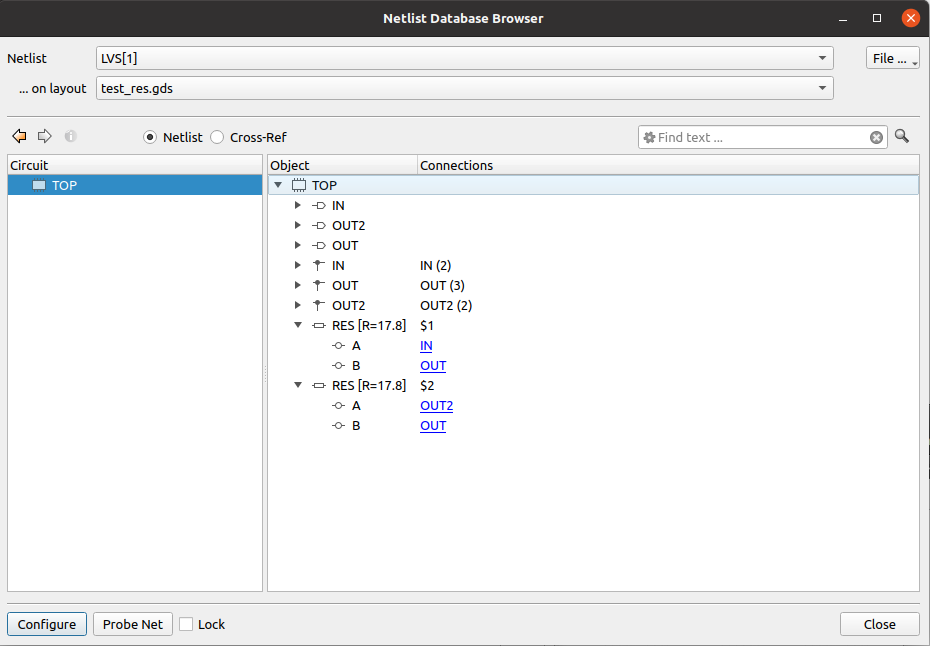In this case, resistors don't get combined as the middle node is a pin.

When I remove the "OUT" labels, this pin is gone and I get a nice combination of the resistory in series: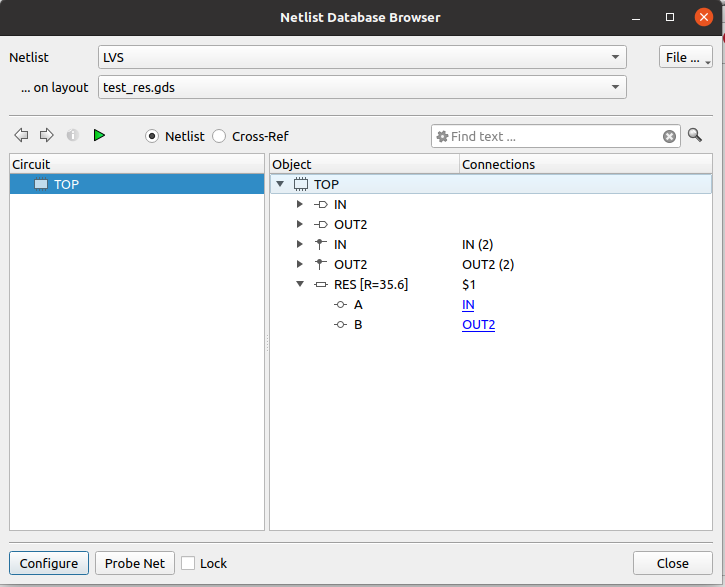So my conclusion is that it's working.

In your previous case, I think the problem was that you shortened the resistors by connecting the resistor layer (which is essentially the body) and the contacts. This makes the body a conductive layer which shorts everything:

``````connect(res_layer,contact_layer)
``````

This is also the reason why "R\$1" in your right netlist is connected to net "1" with both terminals.